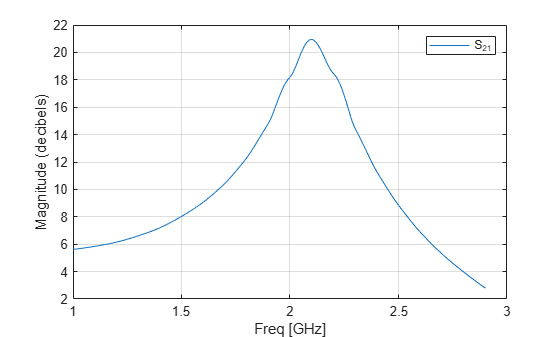# rfdata.data

Store result of circuit object analysis

## Description

Use the `data` class to store S-parameters, noise figure in decibels, and frequency-dependent, third-order output (OIP3) intercept points.

There are three ways to create an `rfdata.data` object:

• You can construct it by specifying its properties from workspace data using the `rfdata.data` constructor.

• You can create it from file data using the `read` method.

• You can perform frequency domain analysis of a circuit object using the `analyze` method, and RF Toolbox™ software stores the results in an `rfdata.data` object.

## Creation

### Syntax

``h = rfdata.data``
``h = rfdata.data('Property1',value1,'Property2',value2,...)``

### Description

example

````h = rfdata.data`returns a data object whose properties all have their default values.```
````h = rfdata.data('Property1',value1,'Property2',value2,...)` sets properties using one or more name-value pairs. You can specify multiple name-value pairs. Enclose each property name in a quote```

## Properties

expand all

Frequency data for the S-parameters in the `S-Parameters` property, specified as a M-element vector in hertz. The values must be positive and correspond to the order of the S-parameters. By default, this property is empty.

Data Types: `double`

Group delay data calculated at each frequency, specified as a M-element vector in seconds. By default, this property is empty.

Data Types: `double`

Interpolation method used in `rfdata.data`, specified as a `1-by-N` character array of the following values:

MethodDescription
`Linear` (default)Linear interpolation
`Spline`Cubic spline interpolation
`Cubic`Piecewise cubic Hermite interpolation

Data Types: `char`

Noise figure, specified as a scalar in dB. `'NF'` is the amount of noise relative to noise temperature of 290 degrees kelvin. The default value is zero indicating a noiseless system.

Data Types: `function_handle`

Output third-order intercept, specified as a scalar in watts. This property represents the hypothetical output signal level at which the third-order tones would reach the same amplitude level as the desired input tones. The default value is `Inf`.

Data Types: `double`

S-parameter data, specified as a `2-by-2-by-M` array. `M` is the number of frequencies at which the network parameters are specified. By default, this property is empty.

Data Types: `double`

Reference impedance, specified as a scalar in ohms. The default value is `50` ohms.

Data Types: `double`

Load impedance, specified as a scalar in ohms. The default value is `50` ohms.

Data Types: `double`

Source impedance, specified as a scalar in ohms. The default value is `50` ohms.

Data Types: `double`

Object name, specified as a `1-by-N` character array or string. This is a read-only property.

Data Types: `char`

## Object Functions

 `analyze` Analyze RFCKT object in frequency domain `calculate` Calculate specified parameters for rfckt objects or rfdata objects `circle` Draw circles on Smith Chart `extract` Extract specified network parameters from rfckt object or data object `listformat` List valid formats for specified circuit object parameter `listparam` List valid parameters for specified circuit object `loglog` Plot specified circuit object parameters using log-log scale `plot` Plot circuit object parameters on X-Y plane `plotyy` Plot parameters of RF circuit or RF data on X-Y plane with two Y-axes `getop` Display operating conditions `polar` Plot specified object parameters on polar coordinates `semilogx` Plot RF circuit object parameters using log scale for x-axis `semilogy` Plot RF circuit object parameters using log scale for y-axis `smith` Plot circuit object parameters on Smith chart `write` Write RF data from circuit or data object to file `getz0` Calculate characteristic impedance of RFCKT transmission line object `read` Read RF data from file to new or existing circuit or data object `restore` Restore data to original frequencies `getop` Display operating conditions `groupdelay` Group delay of S-parameter object or RF filter object or RF Toolbox circuit object

## Examples

collapse all

```file = 'default.s2p'; h = read(rfdata.data,file); % Read file into data object. figure plot(h,'s21','db'); % Plot dB(S21) in XY plane.```## Version History

Introduced in R2009a